3. Analysing species distribution data¶

Introduction¶

In this case-study I show some techniques that can be used to analyze species distribution data with R. Before going through this document you should at least be somewhat familiar with R and spatial data manipulation in R. This document is based on an analysis of the distribution of wild potato species by Hijmans and Spooner (2001). Wild potatoes (Solanaceae; Solanum sect. Petota are relatives of the cultivated potato. There are nearly 200 different species that occur in the Americas.

Import and prepare data¶

The data we will use is available in the rspatial package. First install that from github, using devtools.

if (!require("rspatial")) devtools::install_github('rspatial/rspatial')

The extracted file is a tab delimited text file. Normally, you would read such a file with something like:

f <- system.file("WILDPOT.txt", package="rspatial")
f
##  "C:/soft/R/R-3.6.0/library/rspatial/WILDPOT.txt"
## Error in read.table(f, header = TRUE): more columns than column names

But that does not work in this case because some lines are incomplete. So we have to resort to some more complicated tricks.

# read all lines using UTF-8 encoding
d <- readLines(f, encoding='UTF-8')
# split each line into elements using the tabs
dd <- strsplit(d, '\t')
# show that the number of elements varies
table(sapply(dd, length))
##
##   18   19   20   21   22
##  300 1372  170 1511 1647
# function to complete each line to 22 items
fun <- function(x) {
r <- rep("", 22)
r[1:length(x)] <- x
r
}
# apply function to each element of the list
ddd <- lapply(dd, fun)
# row bind all elements (into a matrix)
v <- do.call(rbind, ddd)
##      [,1]  [,2]       [,3]       [,4]    [,5]    [,6]    [,7]    [,8]
## [1,] "ID"  "COLNR"    "DATE"     "LongD" "LongM" "LongS" "LongH" "LatD"
## [2,] "55"  "OKA 3901" "19710405" "65"    "45"    "0"     "W"     "22"
## [3,] "16"  "OKA 3920" "19710406" "66"    "6"     "0"     "W"     "21"
## [4,] "204" "HOF 1848" "19710305" "65"    "5"     "0"     "W"     "22"
## [5,] "545" "OKA 4015" "19710411" "66"    "15"    "0"     "W"     "22"
## [6,] "549" "OKA 4026" "19710411" "66"    "12"    "0"     "W"     "22"
##      [,9]   [,10]  [,11]  [,12]              [,13]       [,14]     [,15]
## [1,] "LatM" "LatS" "LatH" "SPECIES"          "SCODE_NEW" "SUB_NEW" "SP_ID"
## [2,] "8"    "0"    "S"    "S. acaule Bitter" "acl"       "ACL"     "1"
## [3,] "53"   "0"    "S"    "S. acaule Bitter" "acl"       "ACL"     "1"
## [4,] "16"   "0"    "S"    "S. acaule Bitter" "acl"       "ACL"     "1"
## [5,] "32"   "0"    "S"    "S. acaule Bitter" "acl"       "ACL"     "1"
## [6,] "30"   "0"    "S"    "S. acaule Bitter" "acl"       "ACL"     "1"
##      [,16]       [,17]   [,18]
## [2,] "ARGENTINA" "Jujuy" "Yavi"
## [3,] "ARGENTINA" "Jujuy" "Santa Catalina"
## [4,] "ARGENTINA" "Salta" "Santa Victoria"
## [5,] "ARGENTINA" "Jujuy" "Rinconada"
## [6,] "ARGENTINA" "Jujuy" "Rinconada"
##      [,19]                                                 [,20]   [,21]
## [1,] "LOCALITY"                                            "PLRV1" "PLRV2"
## [2,] "Tafna."                                              "R"     "R"
## [3,] "10 km W of Santa Catalina."                          "S"     "R"
## [4,] "53 km E of Cajas."                                   "S"     "R"
## [5,] "\"Near Abra de Fundiciones, 10 km S of Rinconada.\"" "S"     "R"
## [6,] "8 km SW of Fundiciones."                             "S"     "R"
##      [,22]
## [1,] "FROST"
## [2,] "100"
## [3,] "100"
## [4,] "100"
## [5,] "100"
## [6,] "100"
#set the column names and remove them from the data
colnames(v) <- v[1,]
v <- v[-1,]
# coerce into a data.frame and change the type of some variables
# to numeric (instead of character)
v <- data.frame(v, stringsAsFactors=FALSE)

The coordinate data is in degrees, minutes, seconds (in separate columns, fortunately), so we need to compute longitude and latitude as single numbers.

# first coerce character values to numbers
for (i in c('LongD', 'LongM', 'LongS', 'LatD', 'LatM', 'LatS')) {
v[, i] <- as.numeric(v[,i])
}
v$lon <- -1 * (v$LongD + v$LongM / 60 + v$LongS / 3600)
v$lat <- v$LatD + v$LatM / 60 + v$LatS / 3600
# Southern hemisphere gets a negative sign
v$lat[v$LatH == 'S'] <- -1 * v$lat[v$LatH == 'S']
##    ID     COLNR     DATE LongD LongM LongS LongH LatD LatM LatS LatH
## 1  55  OKA 3901 19710405    65    45     0     W   22    8    0    S
## 2  16  OKA 3920 19710406    66     6     0     W   21   53    0    S
## 3 204  HOF 1848 19710305    65     5     0     W   22   16    0    S
## 4 545  OKA 4015 19710411    66    15     0     W   22   32    0    S
## 5 549  OKA 4026 19710411    66    12     0     W   22   30    0    S
## 6 551 OKA 4030A 19710411    66    12     0     W   22   28    0    S
##            SPECIES SCODE_NEW SUB_NEW SP_ID   COUNTRY  ADM1           ADM2
## 1 S. acaule Bitter       acl     ACL     1 ARGENTINA Jujuy           Yavi
## 2 S. acaule Bitter       acl     ACL     1 ARGENTINA Jujuy Santa Catalina
## 3 S. acaule Bitter       acl     ACL     1 ARGENTINA Salta Santa Victoria
## 4 S. acaule Bitter       acl     ACL     1 ARGENTINA Jujuy      Rinconada
## 5 S. acaule Bitter       acl     ACL     1 ARGENTINA Jujuy      Rinconada
## 6 S. acaule Bitter       acl     ACL     1 ARGENTINA Jujuy      Rinconada
##                                            LOCALITY PLRV1 PLRV2 FROST
## 1                                            Tafna.     R     R   100
## 2                        10 km W of Santa Catalina.     S     R   100
## 3                                 53 km E of Cajas.     S     R   100
## 4 "Near Abra de Fundiciones, 10 km S of Rinconada."     S     R   100
## 5                           8 km SW of Fundiciones.     S     R   100
## 6                "Salveayoc, 5 km SW of Rinconada."     S     R   100
##         lon       lat
## 1 -65.75000 -22.13333
## 2 -66.10000 -21.88333
## 3 -65.08333 -22.26667
## 4 -66.25000 -22.53333
## 5 -66.20000 -22.50000
## 6 -66.20000 -22.46667

Get a SpatialPolygonsDataFrame with most of the countries of the Americas.

library(raster)
library(rspatial)
cn <- sp_data('pt_countries')
proj4string(cn) <- CRS("+proj=longlat +datum=WGS84")
class(cn)
##  "SpatialPolygonsDataFrame"
## attr(,"package")
##  "sp"

Make a quick map

plot(cn, xlim=c(-120, -40), ylim=c(-40,40), axes=TRUE)
points(v$lon, v$lat, cex=.5, col='red')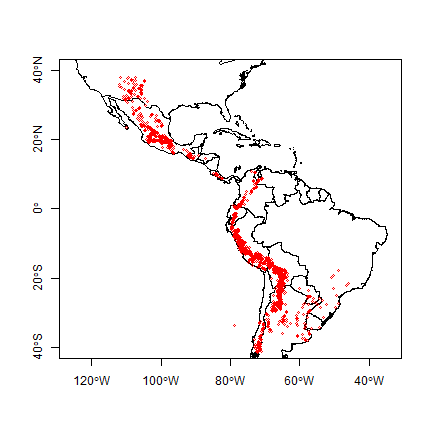And create a SpatialPointsDataFrame for the potato data with the formula approach

sp <- v
coordinates(sp) <- ~lon + lat
proj4string(sp) <- CRS("+proj=longlat +datum=WGS84")

Alternatively, you can do

sp <- SpatialPoints( v[, c('lon', 'lat')],
proj4string=CRS("+proj=longlat +datum=WGS84") )
sp <- SpatialPointsDataFrame(sp, v)

Summary statistics¶

We are first going to summarize the data by country. We can use the country variable in the data, or extract that from the countries SpatialPolygonsDataFrame.

table(v$COUNTRY) ## ## ARGENTINA BOLIVIA BRAZIL CHILE COLOMBIA ## 1474 985 17 100 107 ## COSTA RICA ECUADOR GUATEMALA HONDURAS Mexico ## 24 138 59 1 2 ## MEXICO PANAMA PARAGUAY Peru PERU ## 843 13 19 1 1043 ## UNITED STATES URUGUAY VENEZUELA ## 157 4 12 # note Peru and PERU v$COUNTRY <- toupper(v$COUNTRY) table(v$COUNTRY)
##
##     ARGENTINA       BOLIVIA        BRAZIL         CHILE      COLOMBIA
##          1474           985            17           100           107
##    COSTA RICA       ECUADOR     GUATEMALA      HONDURAS        MEXICO
##            24           138            59             1           845
##        PANAMA      PARAGUAY          PERU UNITED STATES       URUGUAY
##            13            19          1044           157             4
##     VENEZUELA
##            12
# same fix for the SpatialPointsDataFrame
sp$COUNTRY <- toupper(sp$COUNTRY)

Below we determine the country using a spatial query, using the “over” function.

ov <- over(sp, cn)
colnames(ov) <- 'name'
##        name
## 1 ARGENTINA
## 2 ARGENTINA
## 3 ARGENTINA
## 4 ARGENTINA
## 5 ARGENTINA
## 6 ARGENTINA
v <- cbind(v, ov)
table(v$COUNTRY) ## ## ARGENTINA BOLIVIA BRAZIL CHILE COLOMBIA ## 1474 985 17 100 107 ## COSTA RICA ECUADOR GUATEMALA HONDURAS MEXICO ## 24 138 59 1 845 ## PANAMA PARAGUAY PERU UNITED STATES URUGUAY ## 13 19 1044 157 4 ## VENEZUELA ## 12 This table is similar to the previous table, but it is not the same. Let’s find the records that are not in the same country according to the original data and the spatial query. # some fixes first # apparantly in the ocean (small island missing from polygon data) v$name[is.na(v$name)] <- '' # some spelling differenes v$name[v$name=="UNITED STATES, THE"] <- "UNITED STATES" v$name[v$name=="BRASIL"] <- "BRAZIL" i <- which(toupper(v$name) != v$COUNTRY) i ##  581 582 1367 1617 1635 1951 1952 1953 1954 2804 2805 2855 2856 3223 ##  3525 plot(cn, xlim=c(-120, -40), ylim=c(-40,40), axes=TRUE) points(sp, cex=.25, pch='+', col='blue') points(sp[i,], col='red', pch='x', cex=1.5)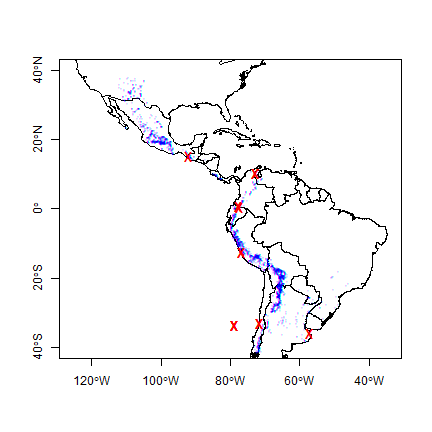All observations that are in a different country than their attribute data suggests are very close to an international border, or in the water. That suggests that the coordinates of the potato locations are not very precise (or the borders are inexact). Otherwise, this is reassuring (and a-typical). There are often are several inconsistencies, and it can be hard to find out whether the locality coordinates are wrong or whether the borders are wrong; but further inspection is warranted in those cases. We can compute the number of species for each country. spc <- tapply(v$SPECIES, sp$COUNTRY, function(x)length(unique(x)) ) spc <- data.frame(COUNTRY=names(spc), nspp = spc) # merge with country SpatialPolygonsDataFrame cn <- merge(cn, spc, by='COUNTRY') print(spplot(cn, 'nspp', col.regions=rev(terrain.colors(25))))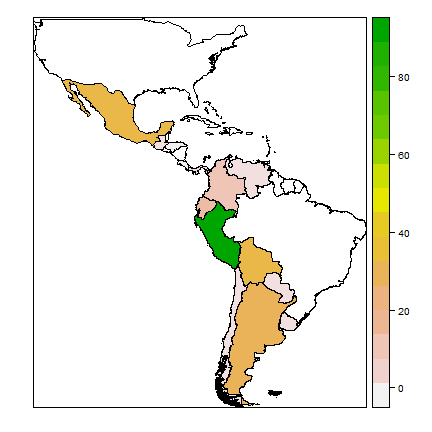The map shows that Peru is the country with most potato species, followed by Bolivia and Mexico. We can also tabulate the number of occurrences of each species by each country. tb <- table(v[ c('COUNTRY', 'SPECIES')]) # a big table dim(tb) ##  16 195 # show two columns tb[,2:3] ## SPECIES ## COUNTRY S. acaule Bitter S. achacachense C<U+643C><U+3E66>rdenas ## ARGENTINA 238 0 ## BOLIVIA 114 8 ## BRAZIL 0 0 ## CHILE 0 0 ## COLOMBIA 0 0 ## COSTA RICA 0 0 ## ECUADOR 0 0 ## GUATEMALA 0 0 ## HONDURAS 0 0 ## MEXICO 0 0 ## PANAMA 0 0 ## PARAGUAY 0 0 ## PERU 52 0 ## UNITED STATES 0 0 ## URUGUAY 0 0 ## VENEZUELA 0 0 Because the countries have such different sizes and shapes, the comparison is not fair (larger countries will have more species, on average, than smaller countries). Some countries are also very large, hiding spatial variation. The map the number of species, it is in most cases better to use a raster (grid) with cells of equal area, and that is what we will do next. Projecting spatial data¶ To use a raster with equal-area cells, the data need to be projected to an equal-area coordinate reference system (CRS). If the longitude/latitude date were used, cells of say 1 square degree would get smaller as you move away from the equator: think of the meridians (vertical lines) on the globe getting closer to each other as you go towards the poles. For small areas, particularly if they only span a few degrees of longitude, UTM can be a good CRS, but it this case we will use a CRS that can be used for a complete hemisphere: Lambert Equal Area Azimuthal. For this CRS, you must choose a map origin for your data. This should be somewhere in the center of the points, to minimize the distance (and hence distortion) from any point to the origin. In this case, a reasonable location is (-80, 0). library(rgdal) # "proj.4" notation of CRS projection(cn) <- "+proj=longlat +datum=WGS84" # the CRS we want laea <- CRS("+proj=laea +lat_0=0 +lon_0=-80") clb <- spTransform(cn, laea) pts <- spTransform(sp, laea) plot(clb, axes=TRUE) points(pts, col='red', cex=.5)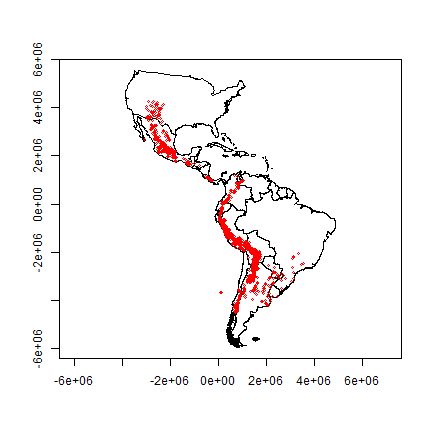Note that the shape of the countries is now much more similar to their shape on a globe than before we projected You can also see that the coordinate system has changed by looking at the numbers of the axes. These express the distance from the origin (-80, 0) in meters. Species richness¶ Let’s determine the distribution of species richness using a raster. First we need an empty ‘template’ raster that has the correct extent and resolution. Here I use 200 by 200 km cells. r <- raster(clb) # 200 km = 200000 m res(r) <- 200000 Now compute the number of observations and the number of species richness for each cell. rich <- rasterize(pts, r, 'SPECIES', function(x, ...) length(unique(na.omit(x)))) plot(rich) plot(clb, add=TRUE)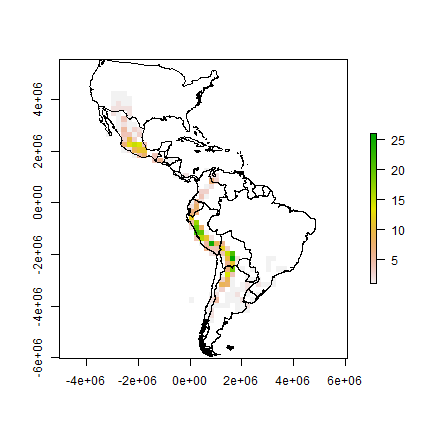Now we make a raster of the number of observations. obs <- rasterize(pts, r, field='SPECIES', fun=function(x, ...)length((na.omit(x))) ) plot(obs) plot(clb, add=TRUE)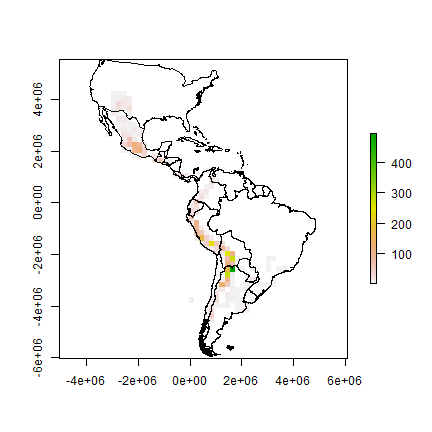A cell by cell comparison of the number of species and the number of observations. plot(obs, rich, cex=1, xlab='Observations', ylab='Richness')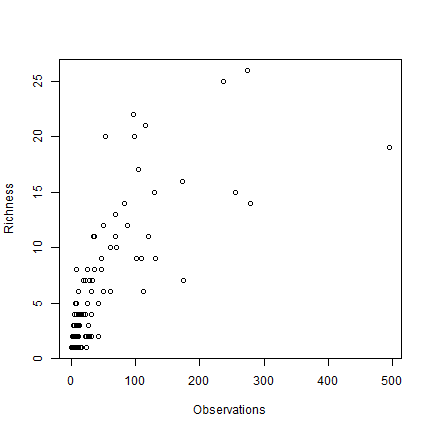Clearly there is an association between the number of observations and the number of species. It may be that the number of species in some places is inflated just because more research was done there. The problem is that this association will almost always exist. When there are only few species in an area, researchers will not continue to go there to increase the number of (redundant) observations. However, in this case, the relationship is not as strong as it can be, and there is a clear pattern in species richness maps, it is not characterized by sudden random like changes in richness (it looks like there is spatial autocorrelation, which is a good thing). Ways to correct for this ‘collector-bias’ include the use of techniques such as ‘rarefaction’ and ‘richness estimators’. There are often gradients of species richness over latitude and altitude. Here is how you can make a plot of the latitudinal gradient in species richness. d <- v[, c('lat', 'SPECIES')] d$lat <- round(d$lat) g <- tapply(d$SPECIES, d$lat, function(x) length(unique(na.omit(x))) ) plot(names(g), g) # moving average lines(names(g), movingFun(g, 3))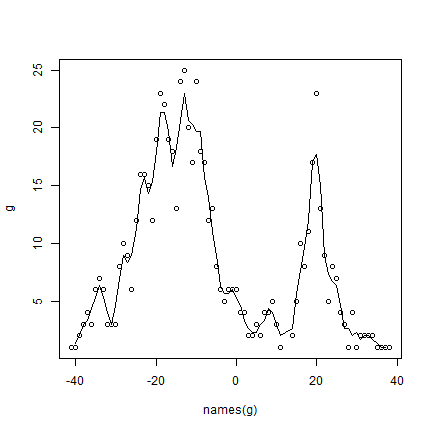** Question ** The distribution of species richness has two peaks. What would explain the low species richness between -5 and 15 degrees? Range size¶ Let’s estimate range sizes of the species. Hijmans and Spooner use two ways: (1) maxD, the maximum distance between any pair of points for a species, and CA50 the total area covered by circles of 50 km around each species. Here, I also add the convex hull. I am using the projected coordinates, but it is also possible to compute these things from the original longitude/latitude data. # get the (Lambert AEA) coordinates from the SpatialPointsDataFrame xy <- coordinates(pts) # list of species sp <- unique(pts$SPECIES)

Compute maxD for each species

maxD <- vector(length=length(sp))
for (s in 1:length(sp)) {
# get the coordinates for species 's'
p <- xy[pts$SPECIES == sp[s], ] # distance matrix d <- as.matrix(dist(p)) # ignore the distance of a point to itself diag(d) <- NA # get max value maxD[s] <- max(d, na.rm=TRUE) } # Note the typical J shape plot(rev(sort(maxD))/1000, ylab='maxD (km)')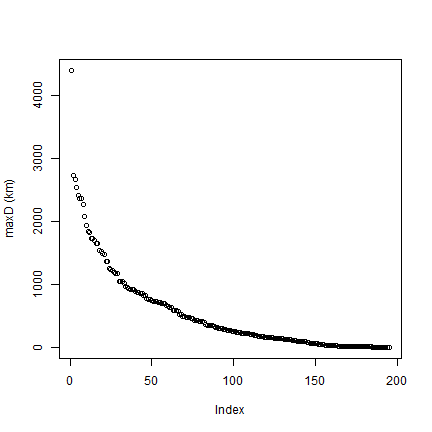Compute CA library(dismo) library(rgeos) CA <- vector(length=length(sp)) for (s in 1:length(sp)) { p <- xy[pts$SPECIES == sp[s], ,drop=FALSE]
# run "circles" model
m <- circles(p, d=50000, lonlat=FALSE)
CA[s] <- area(polygons(m))
}
# standardize to the size of one circle
CA <- CA / (pi * 50000^2)
plot(rev(sort(CA)), ylab='CA50')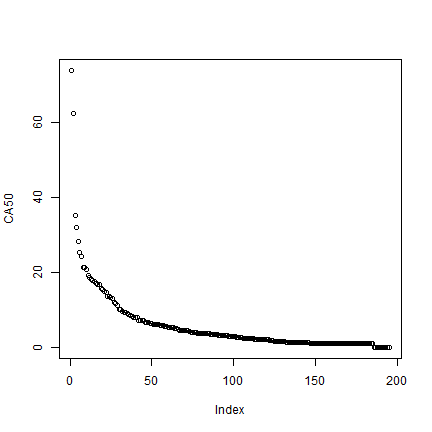Make convex hull range polygons

hull <- list()
for (s in 1:length(sp)) {
p <- unique(xy[pts$SPECIES == sp[s], ,drop=FALSE]) # need at least three points for hull if (nrow(p) > 3) { h <- convHull(p, lonlat=FALSE) pol <- polygons(h) hull[[s]] <- pol } } Plot the hulls. First remove the empty hulls (you cannot make a hull if you do not have at least three points). # which elements are NULL i <- which(!sapply(hull, is.null)) h <- hull[i] # combine them hh <- do.call(bind, h) plot(hh)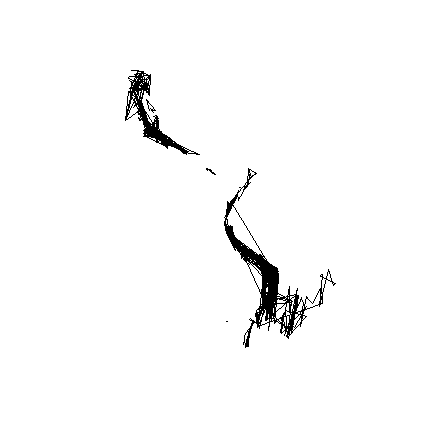Get the area for each hull, taking care of the fact that some are NULL. ahull <- sapply(hull, function(i) ifelse(is.null(i), 0, area(i))) plot(rev(sort(ahull))/1000, ylab='Area of convex hull')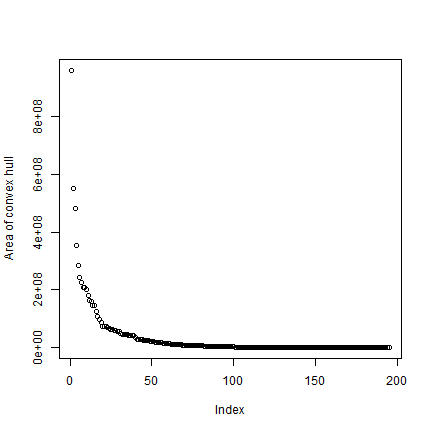Compare all three measures d <- cbind(maxD, CA, ahull) pairs(d)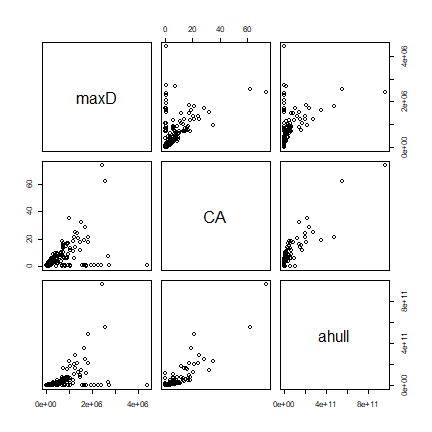Exercises¶ Exercise 1. Mapping species richness at different resolutions¶ Make maps of the number of observations and of species richness at 50, 100, 250, and 500 km resolution. Discuss the differences. Exercise 2. Mapping diversity¶ Make a map of Shannon Diversity H for the potato data, at 200 km resolution. 1. First make a function that computes Shannon Diversity (H) from a vector of species names H = -SUM(p * ln(p)) Where p is proportion of each species To get p, you can do vv <- as.vector(table(v$SPECIES)) p <- vv / sum(vv)

1. now use the function

Exercise 3. Mapping traits¶

There is information about two traits in the data set in field PRLV (tolerance to Potato Leaf Roll Virus) and frost (frost tolerance). Make a map of average frost tolerance.